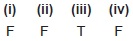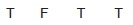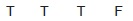# User Forum

Subject :IMO    Class : Class 3

State 'T' for true and 'F' for false.
(i) The smallest three-digit odd number is 999.
(ii) 3000 is equal to 30 tens.
(iii) The place value of 3 in eight thousand two hundred thirty four is tens.
(iv) 1000 + 1 is the greatest three-digit odd number.

ABCD